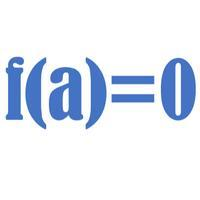# Factor and Remainder TheoremThe factor theorem is a special case of the remainder theorem, in that (x-a) is a factor if and only if P(a)=0

To access the contents of this site, you need to log in or subscribe